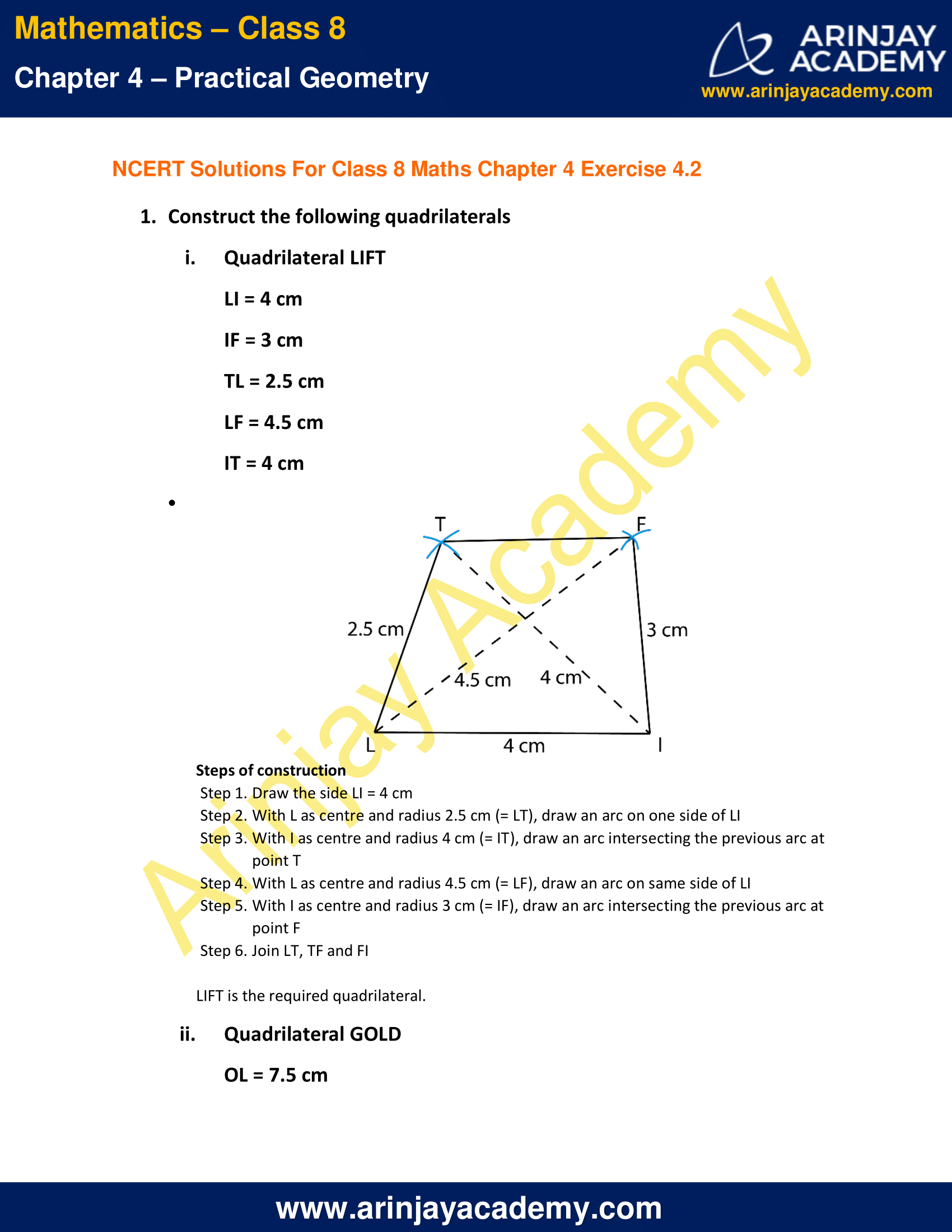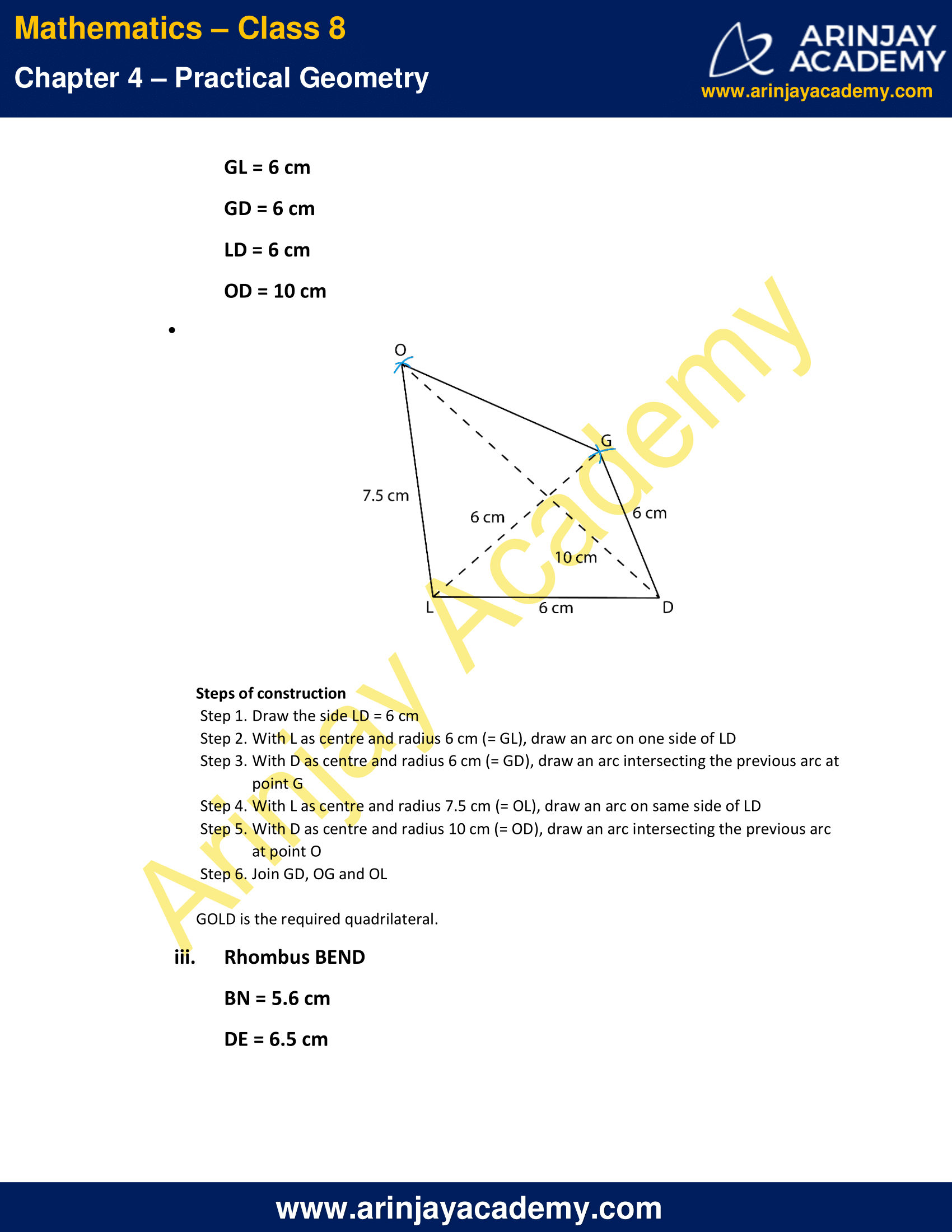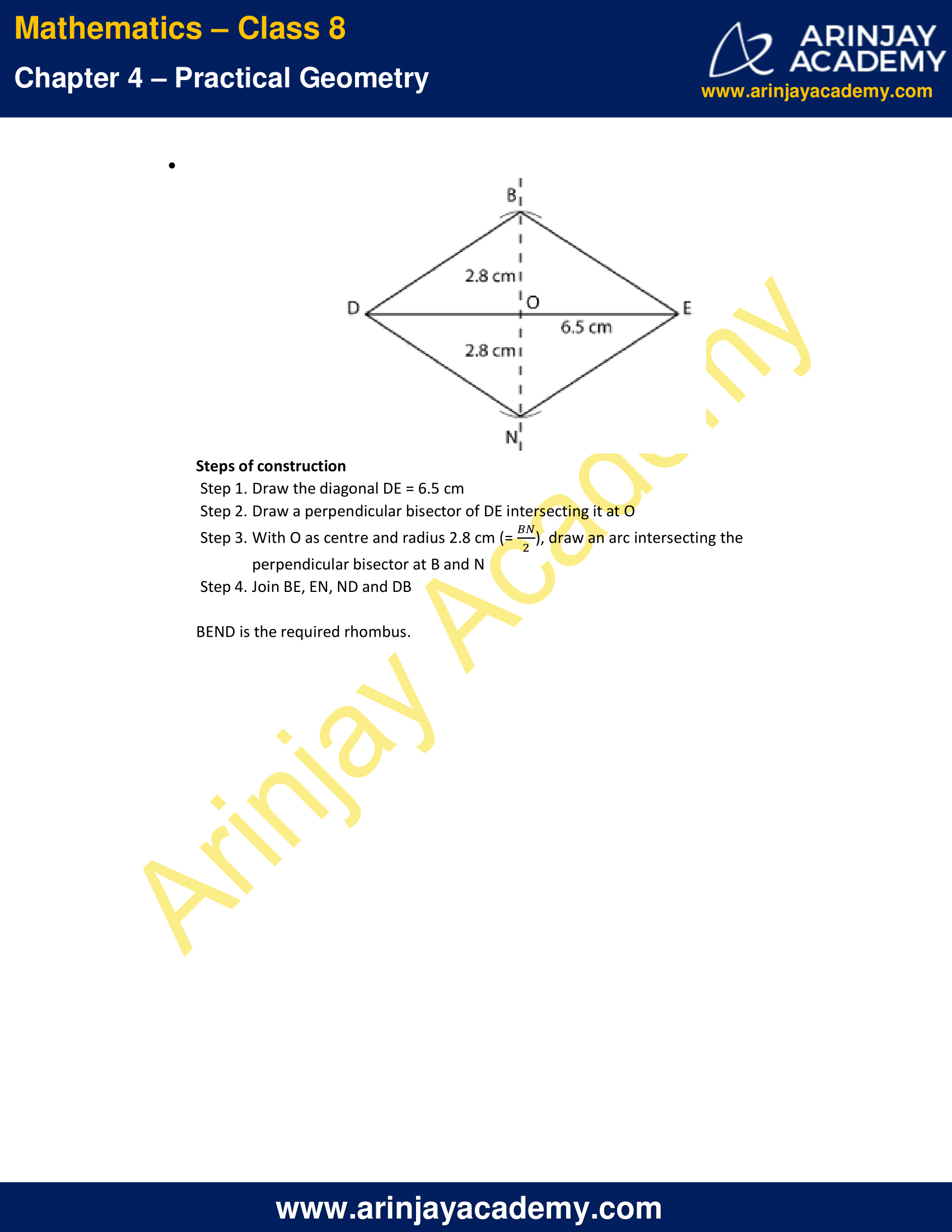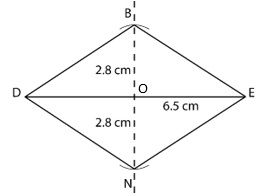# NCERT Solutions for Class 8 Maths Chapter 4 Exercise 4.2 – Practical Geometry

NCERT Solutions for Class 8 Maths Chapter 4 Exercise 4.2 – Practical Geometry, has been designed by the NCERT to test the knowledge of the student on the topic – Constructing a Quadrilateral – When two diagonals and three sides are given

### NCERT Solutions for Class 8 Maths Chapter 4 Exercise 4.2 – Practical GeometryNCERT Solutions for Class 8 Maths Chapter 4 Exercise 4.2 – Practical Geometry

LI = 4 cm
IF = 3 cm
TL = 2.5 cm
LF = 4.5 cm
IT = 4 cm

Solution:Steps of construction

• Step 1. Draw the side LI = 4 cm
• Step 2. With L as centre and radius 2.5 cm (= LT), draw an arc on one side of LI
• Step 3. With I as centre and radius 4 cm (= IT), draw an arc intersecting the previous arc at point T
• Step 4. With L as centre and radius 4.5 cm (= LF), draw an arc on same side of LI
• Step 5. With I as centre and radius 3 cm (= IF), draw an arc intersecting the previous arc at point F
• Step 6. Join LT, TF and FI

OL = 7.5 cm
GL = 6 cm
GD = 6 cm
LD = 6 cm
OD = 10 cm

Solution:Steps of construction

• Step 1. Draw the side LD = 6 cm
• Step 2. With L as centre and radius 6 cm (= GL), draw an arc on one side of LD
• Step 3. With D as centre and radius 6 cm (= GD), draw an arc intersecting the previous arc at point G
• Step 4. With L as centre and radius 7.5 cm (= OL), draw an arc on same side of LD
• Step 5. With D as centre and radius 10 cm (= OD), draw an arc intersecting the previous arc at point O
• Step 6. Join GD, OG and OL

iii. Rhombus BEND

BN = 5.6 cm
DE = 6.5 cm

Solution:Steps of construction

• Step 1. Draw the diagonal DE = 6.5 cm
• Step 2. Draw a perpendicular bisector of DE intersecting it at O
• Step 3. With O as centre and radius 2.8 cm (=$\cfrac { BN }{ 2 }$), draw an arc intersecting the perpendicular bisector at B and N
• Step 4. Join BE, EN, ND and DB

BEND is the required rhombus.

The next Exercise for NCERT Solutions for Class 8 Maths Chapter 4 Exercise 4.3 – Practical Geometry can be accessed by clicking here

Download NCERT Solutions for Class 8 Maths Chapter 4 Exercise 4.2 – Practical Geometry